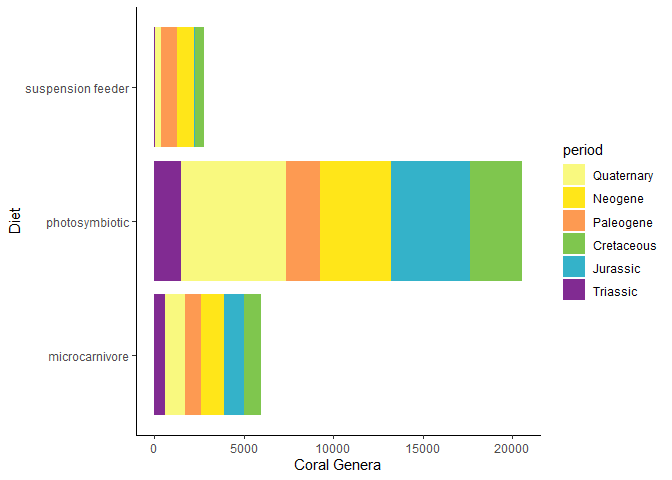# Adding geological timescales to plots

The main function of deeptime is coord_geo(), which functions just like coord_trans() from {ggplot2}. You can use this function to add highly customizable timescales to a wide variety of ggplots. Before we begin, let’s load some necessary packages first.

# Load deeptime
library(deeptime)
library(ggplot2)
library(dplyr)
library(magrittr) # for piping
# Load divDyn for coral data
library(divDyn)
data(corals)
# Load gsloid for oxygen isotope data
library(gsloid)

First, let’s summarize some coral data from the divDyn package to generate a diversity curve that we’ll be able to plot:

# this is not a proper diversity curve but it gets the point across
coral_div <- corals %>%
filter(stage != "") %>%
group_by(period, stage) %>%
summarise(n = n()) %>%
mutate(stage_age = (stages$max_age[match(stage, stages$name)] +
stages$min_age[match(stage, stages$name)]) / 2)

Now, we can plot the data and add a geological timescale. The default behavior is to add the ICS periods to the bottom axis. The data used for this is included in the package (?periods) along with data for ?eons, ?stages, ?epochs, and ?eras. Note that the function assumes that age is decreasing towards the present.

ggplot(coral_div) +
geom_line(aes(x = stage_age, y = n)) +
scale_x_reverse("Age (Ma)") +
ylab("Coral Genera") +
coord_geo(xlim = c(250, 0), ylim = c(0, 1700)) +
theme_classic()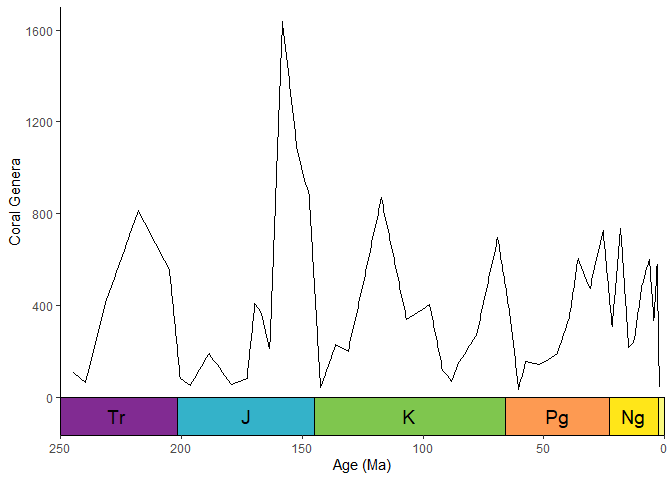### Scales on other axes

You can add the timescale to whichever axis you’d like by setting the pos argument. For example, pos = "left" can be used to put the timescale on the left axis. Also, note that we’re using the “Geomagnetic Polarity Chron” timescale here. This is one of more than 35 regional and global timescales that are available through deeptime from the Macrostrat database (see get_scale_data()).

ggplot(lisiecki2005) +
geom_line(aes(x = d18O, y = Time / 1000), orientation = "y") +
scale_y_reverse("Time (Ma)") +
scale_x_reverse() +
coord_geo(
dat = "Geomagnetic Polarity Chron", xlim = c(6, 2), ylim = c(6, 0),
pos = "left", rot = 90
) +
theme_classic()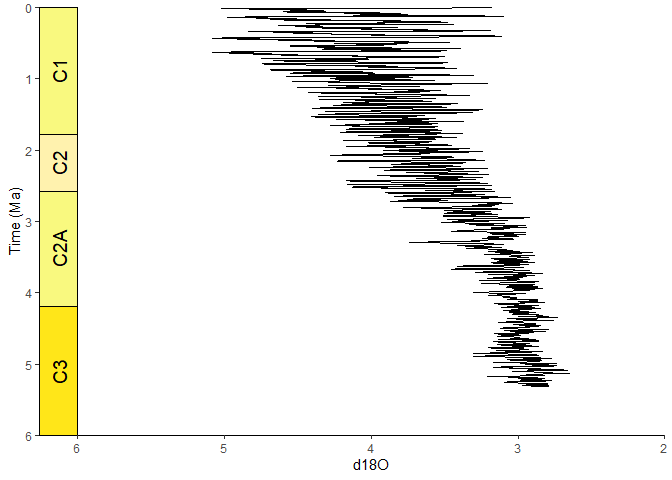You can also add multiple timescales by providing a list for pos. Other arguments can be lists or single values (either of which will be recycled if necessary). In this case we are adding the “Geomagnetic Polarity Chron” timescale to the left axis and the “Planktic foraminiferal Primary Biozones” timescale to the right axis.

# uses the oxygen isotope data from above
ggplot(lisiecki2005) +
geom_line(aes(x = d18O, y = Time / 1000), orientation = "y") +
scale_y_reverse("Time (Ma)") +
scale_x_reverse() +
coord_geo(
dat = list("Geomagnetic Polarity Chron", "Planktic foraminiferal Primary Biozones"),
xlim = c(6, 2), ylim = c(5.5, 0), pos = list("l", "r"),
rot = 90, skip = "PL4", size = list(5, 4)
) +
theme_classic()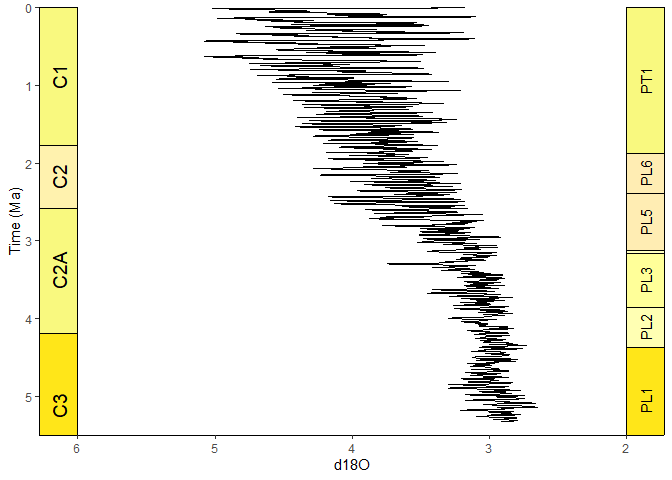### Stack multiple scales

You can even stack multiple timescales on the same side. Scales are added from the inside to the outside. Here we are stacking periods and eras.

# uses the coral diversity data from above
ggplot(coral_div) +
geom_line(aes(x = stage_age, y = n)) +
scale_x_reverse("Age (Ma)") +
ylab("Coral Genera") +
coord_geo(
dat = list("periods", "eras"), xlim = c(250, 0), ylim = c(0, 1700),
pos = list("b", "b"), abbrv = list(TRUE, FALSE)
) +
theme_classic()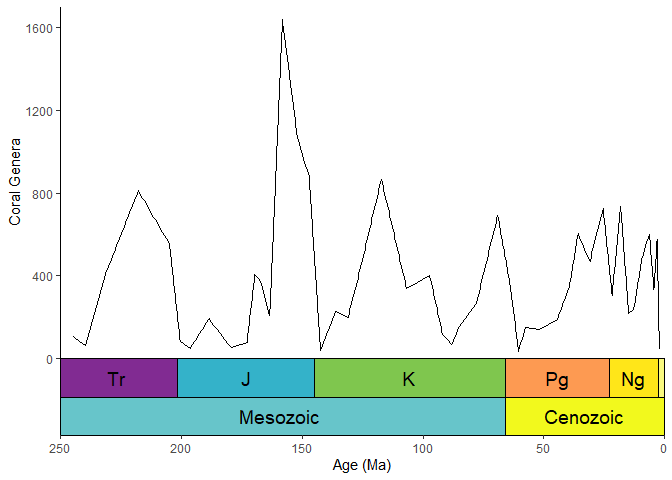### Timescales and faceted plots

coord_geo() synergizes well with faceted plots. You can change on which facets the timescale is plotted by changing the scales argument in facet_wrap(). The default behavior is to only include the timescale on the bottom-most panel. This behavior works similarly with facet_grid().

# uses the coral occurrence data from above
coral_div_diet <- corals %>%
filter(stage != "") %>%
group_by(diet, stage) %>%
summarise(n = n()) %>%
mutate(stage_age = (stages$max_age[match(stage, stages$name)] +
stages$min_age[match(stage, stages$name)]) / 2)

ggplot(coral_div_diet) +
geom_line(aes(x = stage_age, y = n)) +
scale_x_reverse("Age (Ma)") +
ylab("Coral Genera") +
coord_geo(xlim = c(250, 0)) +
theme_classic() +
facet_wrap(~diet, nrow = 3)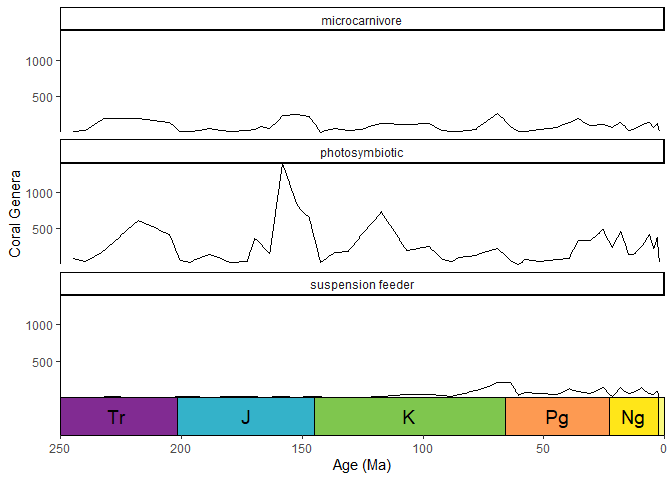By specifying scales = "free_x", you can add a geological time scale to each panel.

ggplot(coral_div_diet) +
geom_line(aes(x = stage_age, y = n)) +
scale_x_reverse("Age (Ma)") +
ylab("Coral Genera") +
coord_geo(xlim = c(250, 0)) +
theme_classic() +
facet_wrap(~diet, nrow = 3, scales = "free_x")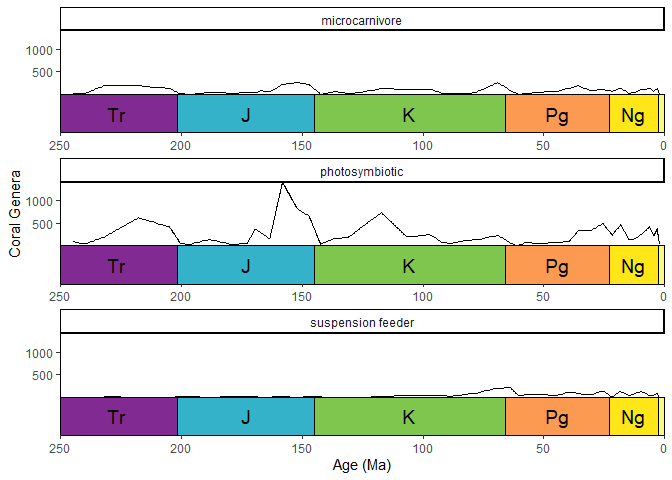### Resize labels to fit inside interval rectangles

coord_geo() can use the {ggfittext} package to resize the interval labels. This can be enabled by setting size to "auto". Additional arguments can be passed to ggfittext::geom_fit_text() as a list using the fittext_args argument.

ggplot(coral_div) +
geom_line(aes(x = stage_age, y = n)) +
scale_x_reverse("Age (Ma)") +
ylab("Coral Genera") +
coord_geo(
dat = "periods", xlim = c(250, 0), ylim = c(0, 1700),
abbrv = FALSE, size = "auto", fittext_args = list(size = 20)
) +
theme_classic()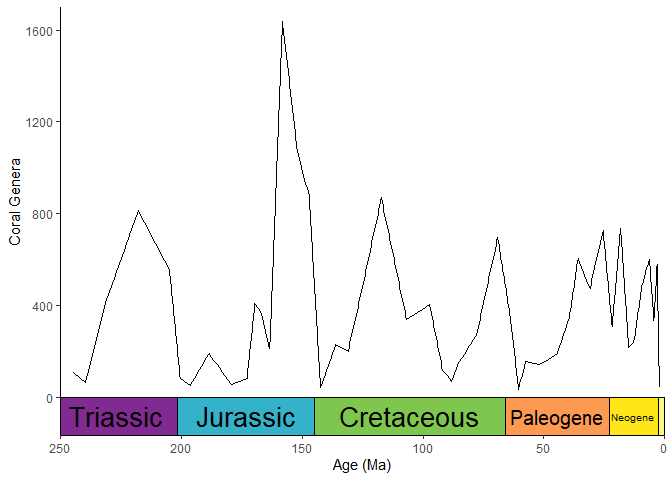## Scales on discrete axes

coord_geo() will automatically detect if your axis is discrete. The categories of the discrete axis (which can be reordered using the limits argument of scale_x_discrete()/scale_y_discrete()) should match the name column of the timescale data (dat). You can use the arguments of theme() and scale_[x/y]_discrete() to optionally remove the labels and tick marks.

# use the coral occurrence data from above
coral_div_dis <- corals %>%
filter(period != "") %>%
group_by(diet, period) %>%
summarise(n = n()) %>%
mutate(period_age = (periods$max_age[match(period, periods$name)] +
periods$min_age[match(period, periods$name)]) / 2) %>%
arrange(-period_age)

ggplot(coral_div_dis) +
geom_col(aes(x = period, y = n, fill = diet)) +
scale_x_discrete("Period",
limits = unique(coral_div_dis$period), labels = NULL, expand = expansion(add = .5) ) + scale_y_continuous(expand = c(0, 0)) + scale_fill_viridis_d("Diet") + ylab("Coral Genera") + coord_geo(expand = TRUE, skip = NULL, abbrv = TRUE) + theme_classic() + theme(axis.ticks.length.x = unit(0, "lines"))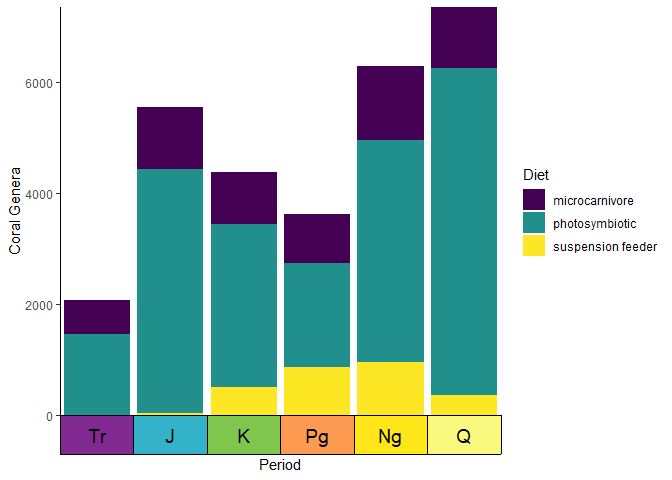### Custom discrete scales You can also supply your own pre-discretized scale data by setting the dat_is_discrete parameter to TRUE. Custom scales should always have name, max_age, and min_age columns. Optional columns include abbr for abbreviations, color for background colors, and lab_color for interval label colors. You can even have one scale with auto-discretized intervals and one scale with pre-discretized intervals. eras_custom <- data.frame( name = c("Mesozoic", "Cenozoic"), max_age = c(0.5, 3.5), min_age = c(3.5, 6.5), color = c("#67C5CA", "#F2F91D") ) ggplot(coral_div_dis) + geom_col(aes(x = period, y = n, fill = diet)) + scale_x_discrete(NULL, limits = unique(coral_div_dis$period), labels = NULL,
) +
scale_y_continuous(expand = c(0, 0)) +
scale_fill_viridis_d("Diet") +
ylab("Coral Genera") +
coord_geo(
dat = list("periods", eras_custom), pos = c("b", "b"), expand = TRUE,
skip = NULL, abbrv = list(TRUE, FALSE), dat_is_discrete = list(FALSE, TRUE)
) +
theme_classic() +
theme(axis.ticks.length.x = unit(0, "lines"))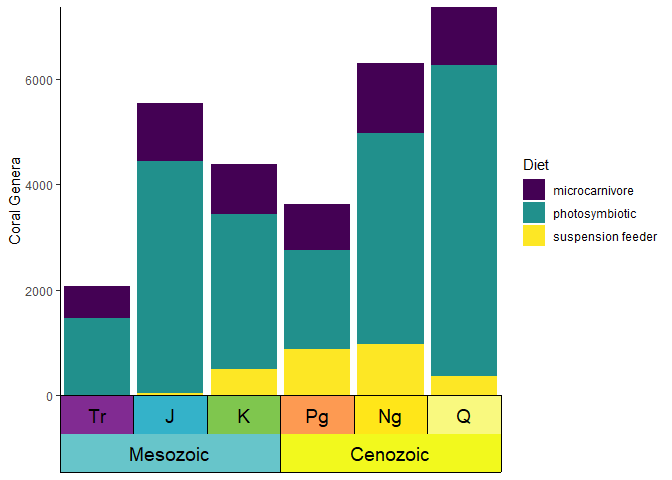## Geological timescale color scales for ggplot

In addition to adding a timescale to a plot, you may also want to color your data based on it’s age. deeptime has scale_color_geo() and scale_fill_geo() for this very purpose! Note that currently these scales only work with discrete data. The default behavior is for the color/fill aesthetic values to match the names of the intervals in dat.

ggplot(coral_div_dis, aes(x = n, y = diet, fill = period)) +
geom_col() +
scale_fill_geo(periods) +
xlab("Coral Genera") +
ylab("Diet") +
theme_classic()